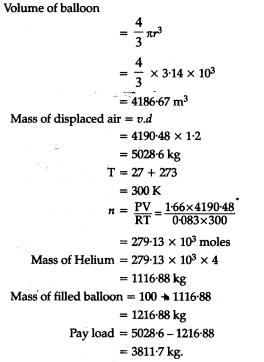# Pay load is defined as the difference between the mass of displaced air and the mass of the balloon

Pay load is defined as the difference between the mass of displaced air and the mass of the balloon. Calculate the pay load when a baloon of radius 10 m, mass 100 kg is filled with helium at 1.66 bar at 27°C. [Density of air = 1.2 kg\${{m}^{-3}}\$, R = 0.083 bar d\${{m}^{3}}\$\${{K}^{-1}}\$\${{mol}^{-1}}\$].